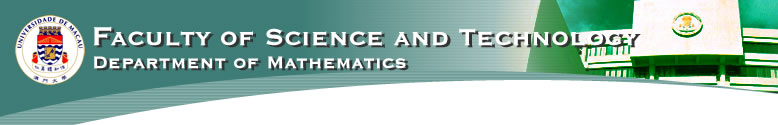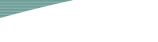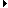HomeNews & ActivitiesDegrees OfferedCoursesAcademic StaffAdministrative StaffAdvisorsContact HourLaboratories and FacilitiesRelated LinksFaculty of Science and Technology

 Credits MATH7001 Functional Analysis 3 MATH7002 Real Analysis 3 6 required electives chosen from the elective table 18 MATH7998 Project Report 6 Total Credits: 30 Elective table for MSc in Mathematics: MATH7003 Advanced Numerical Linear Algebra 3 MATH7004 Advanced Probability and Statistics 3 MATH7005 Clifford Analysis 3 MATH7006 Geometry and Its Applications 3 MATH7007 Mathematical Theory of Computational Intelligence 3 MATH7008 Matrix Analysis 3 MATH7009 Numerical Methods for Differential Equations 3 MATH7010 Partial Differential Equations 3 MATH7011 Reading Course I 3 MATH7012 Reading Course II 3 MATH7013 Stochastic Differential Equations 3 MATH7014 Stochastic Processes 3 MATH7015 Time Series Analysis 3 MATH7016 Topics in Analysis 3 MATH7017 Topics in Geometry 3 MATH7018 Topics in Matrix Analysis 3 MATH7019 Topics in Partial Differential Equations 3 MATH7020 Topics in Probability and Statistics 3 MATH7021 Financial Mathematics 3